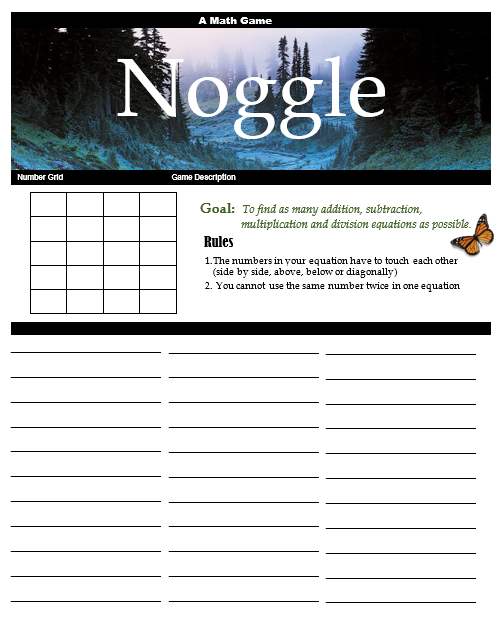## Friday, October 24, 2014

### Noggle: A Math Fact Game

Today's post continues our occasional series about classroom games and tools developed by Yellin Center Learning Specialist Renee Jordan during her years as a classroom teacher.

2 through 8

Curricular Area: Mathematics

Description of Game:
Mastery of math facts is a vital component of math education. Math facts are the building blocks of all other higher level math operations. Therefore, it is important that students have abundant opportunities to practice their math facts. Noggle is an enjoyable math game for students that can be used as an alternative to fact drills and traditional worksheets.

Noggle is customizable across grade levels, since the numbers can be changed to reflect the abilities of the students in your classroom. Be sure when you are picking numbers to fill in your grid that you are able locate multiple math facts. Another way to adapt this game is by the types of equations you ask your students to search for (e.g. addition, subtraction, division or multiplication).

In my classroom, I have used Noggle as an early finisher activity, a morning puzzle, or as one option in a math game lesson. I found that I was using Noggle so often as a start to the day puzzle or early finisher activity that I taped out a grid using painter's tape on a spare whiteboard mounted in my classroom. This also encouraged my students to create their own number grids on our classroom Noggle board that their peers could try and solve. Alternatively, a teaching colleague used open space on one of her bulletin boards to devise her own permanent Noggle board.This is the Noggle Board I used with my students, but you can create your own.

Materials Needed:
One handout per student

Instructions:

1. Draw a game grid on the board

2. Fill in the game board with numbers of your choosing

Tips:
i. Be sure to check your numbers and see that you can find a several equations that your students would be able to locate and solve independently

ii. Example of completed grid with addition, subtraction and multiplication equations

 3 4 9 5 6 2 8 4 9 6 1 7 3 4 7 6

iii   Example answers from the aforementioned grid using the numeral 3 in the top left hand corner (in the shaded cell): 3 +4 = 9; 4+3=9; 9-4=3; 3+6=9; 6+3=9; 9-6=3; 3+2+1=6; 3+4+2=9 3x2=6

3.  Explain the rules of the game to your students and designate the types of equations you would like them to find (e.g. addition, subtraction, division or multiplication).

Tips
i.      I often reference the game Boggle and explain that it is a similar structure but with numbers instead of letters, and that you are finding equations instead of words.
ii.      For the younger grades I will only select addition or subtraction;  for older grades I will challenge students to find the additional equation types.
iii. I always encourage my students to find equations with more than two numbers (e.g. 1+3+4= 8)
iv. I always encourage my students to use the communicative property (e.g. a+b=c and b+a=c)

4.  Highlight the text at the top of the game board where the students can refer to the goal and game rules in case they forget

5. Allow the students time to play the game.

Alignment with Common Core Standards:
• CCSS.MATH.CONTENT.3.OA.C.7: Fluently multiply and divide within 100, using strategies such as the relationship between multiplication and division (e.g., knowing that 8 × 5 = 40, one knows 40 ÷ 5 = 8) or properties of operations. By the end of Grade 3, know from memory all products of two one-digit numbers.